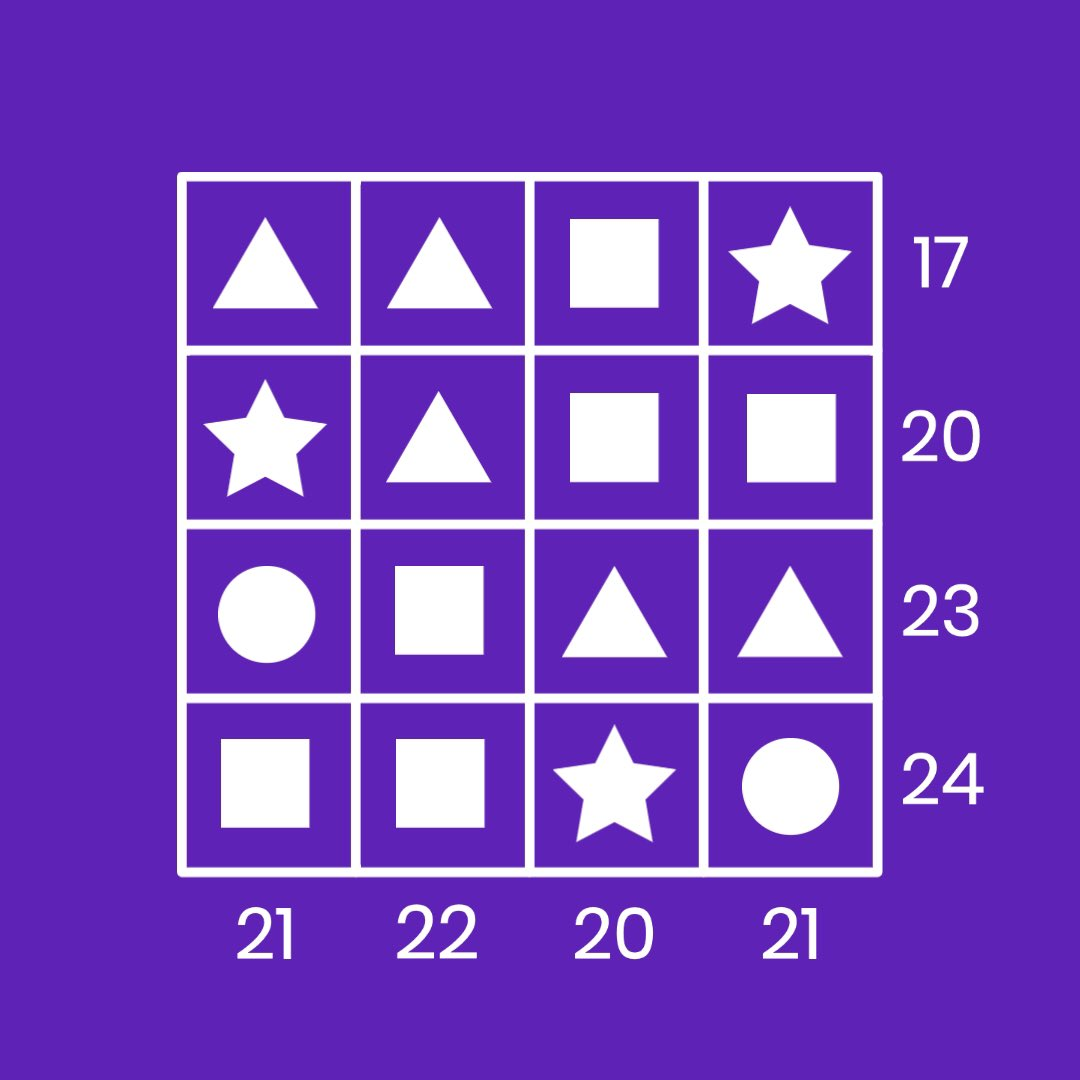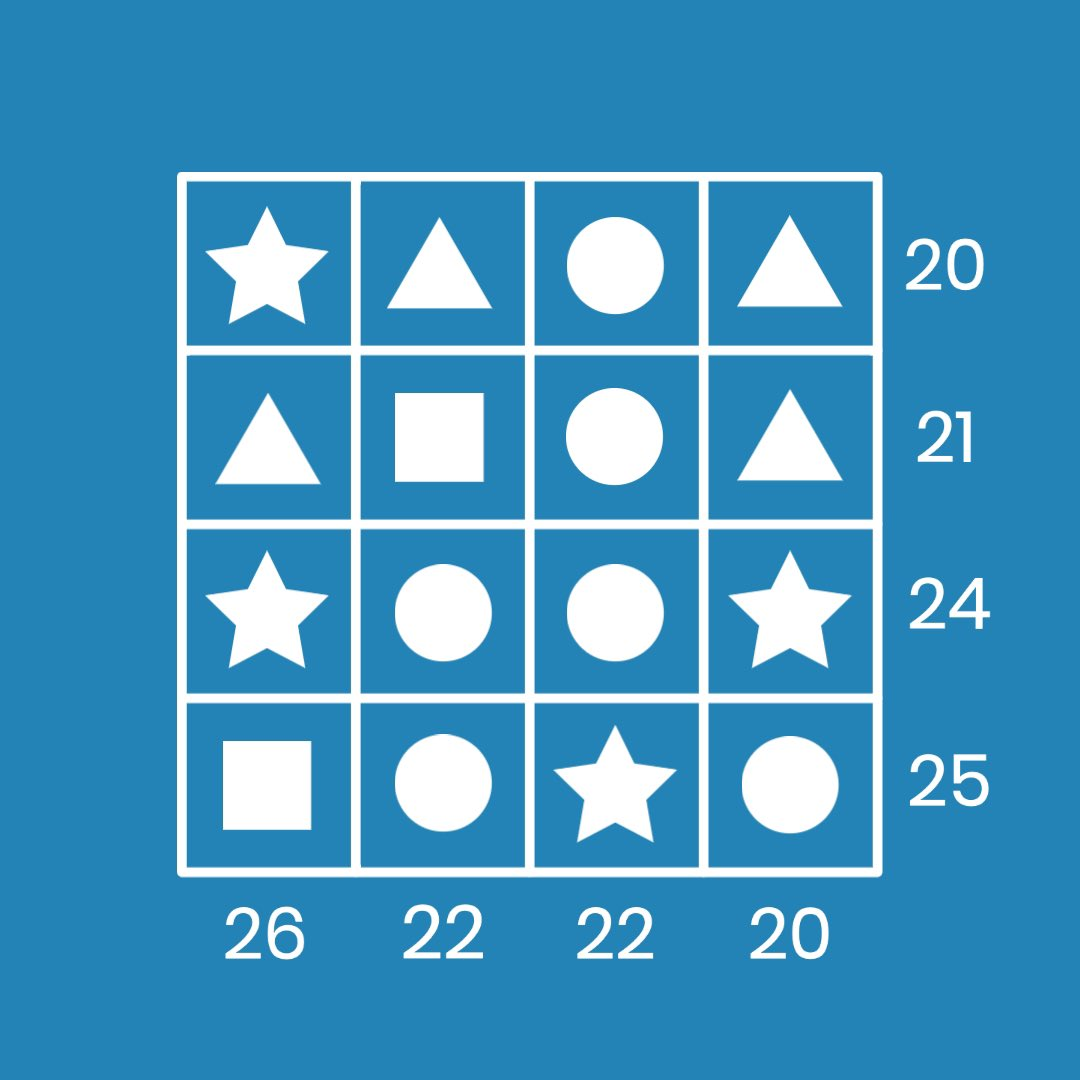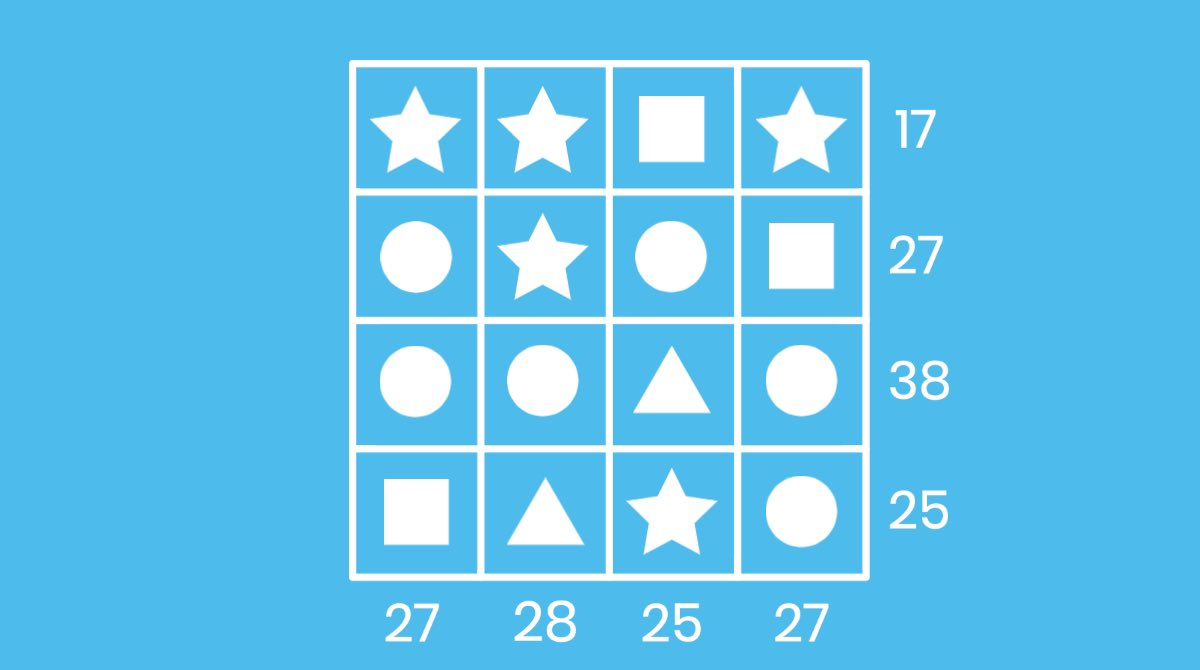Each shape represents a positive whole number from 1-10. The sum total of the shapes in each row/column is displayed at the end of each row/column. Use this knowledge to deduce the numerical value of each shape.

#symbolsofvalue #saturdaysov #puzzles #books #challenge https://t.co/d3KTPEVeNEEach shape represents a positive whole number from 1-10. The sum total of the shapes in each row/column is displayed at the end of each row/column. Use this knowledge to deduce the numerical value of each shape.

#symbolsofvalue #saturdaysov #puzzles #books #challenge https://t.co/LlLt7D8625Each shape represents a positive whole number from 1-10. The sum total of the shapes in each row/column is displayed at the end of each row/column. Use this knowledge to deduce the numerical value of each shape.

#symbolsofvalue #saturdaysov #puzzles #books #challenge https://t.co/aSjy4BGLPBEach shape represents a positive whole number from 1-10. The sum total of the shapes in each row/column is displayed at the end of each row/column. Use this knowledge to deduce the numerical value of each shape.

#symbolsofvalue #saturdaysov #puzzles #books #challenge https://t.co/lLk3IZje6g